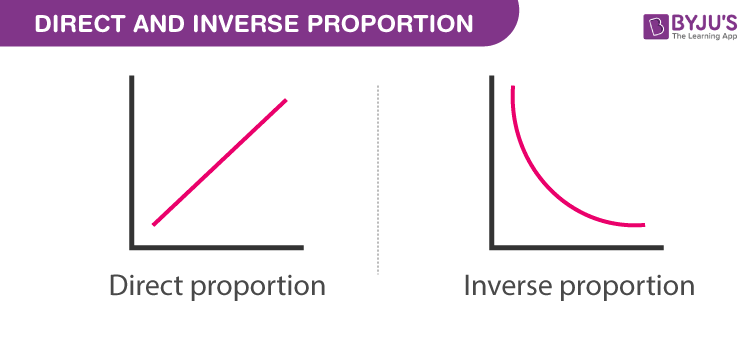# Inversely proportional

When the value of one quantity increases with respect to decrease in other or vice-versa, then they are said to be inversely proportional. It means that the two quantities behave opposite in nature. For example, speed and time are in inverse proportion with each other. As you increase the speed, the time is reduced. The other terms used here for this type of proportion are inverse proportion or varying inversely or inverse variation or reciprocal proportion. Two variables say x and y, which are in inverse proportion are represented as;

x ∝ 1/y or x ∝ y-1

In Mathematics as well as in Physics, we learn about quantities. Some quantities depend upon one another, and such quantities are termed as proportional to one another. In other words, two variables are said to be proportional to each other, if one is changed, then the other is also changed by a fixed amount. This property of variables is known as proportionality. The symbol used to represent the proportionality is “∝.”

There are two types of proportionality of variables. They are:

• Directly Proportional
• Inversely Proportional

Some time we termed these proportionalities as two variables or quantities in direct proportion or inverse proportion.

## Inversely Proportional Meaning

Directly proportional variables are those in which if one variable increases, the other also increases. If one variable decreases, the other decreases in the same proportion. Inversely proportional variables are those in which one variable decreases with the increase in another variable and one variable increases with the decrease in another variable. It is opposite to direct proportion. Two quantities are said to be inversely proportional when one quantity is in direct proportion to the reciprocal of others.## Inversely Proportional Definition

Two variables are called inversely proportional, if and only if the variables are directly proportional to the reciprocal of each other. Or we can say when two variables or quantities are in inverse proportion, then the product of the two variables is equal to a constant value. Ultimately, when the value of one variable increases, then the value of another variable decreases and their product remains constant or unchanged.

### Inversely Proportional Formula

If x and y are two quantities which are in inverse variations, then

x ∝ 1/y

x = k(1/y)

Where “k” is a universally positive constant.

It can also be represented as xy = k

If x and y are in inverse variation and x has two values x1 and x2 corresponding to y having two values y1 and y2 respectively, then by the definition of inverse variation, we have

x1 y1 = x2 y2 = (k)

In this case, it becomes

x1 / x2 = y2 / y1 = k

### Inversely Proportional Example

Question 1: Find A when B = 500, if A is inversely proportional to B, and also given that A = 200, B = 0.6.

Solution:

Given,

Here A is inversely proportional to B

i.e. A = k (1/B)

Where k is a constant and we get,

k = A x B

Given that,

A = 200 and B = 0.6

So, k = 200 x 0.6 = 120

Therefore, k = 120.

From the above equation, we get

k = 120

Now, substitute the value of k to find A

120 = A x 500

A = (120)/(500) = 0.24

Therefore, A = 0.24

Question 2: Suppose x and y are in an inverse proportion such that, when x = 15, then y = 8. Find the value of y when x = 20.

Solution:

Given,

x ∝ 1/y

x = k/y, where k is a constant

or k = xy

Substituting, x = 15 and y = 8, we get;

k = 15 x 8 = 120

Now, when x = 20, then;

20 y = 120

y = 120/20 = 6

That means, when x is increased to 20 then y decreases to 6.

## Frequently Asked Questions – FAQs

Q1

### What is the formula for inversely proportional?

Suppose two variables x and y are inversely proportional; then we can represent them as x ∝ 1/y. That means, if the value of x increases, then the value of y decreases and vice versa.
Q2

### What is the meaning of inversely and directly proportional?

If two variables x, y are inversely proportional, then x ∝ 1/y, that means xy = k (some constant), and if they are directly proportional, then x ∝ y that means x/y = k.
Q3

### What is another word for inversely proportional?

Another word inversely proportional is inverse variation.
Q4

### What is directly proportional?

Two variables, x and y, are called directly proportional if an increase in x or y causes a corresponding increase in the other variable, i.e. y or x, or a decrease in one variable results in a reduction of the other variable. In this case, the product of x and y is equal to a constant value.
Q5

### What is an example of an inverse proportion?

As we know, inverse proportion occurs when a decrease in one quantity or variable causes an increase in another quantity or variable. For example, a reduction of workers’ number increases the number of days to complete the building’s construction.
Test your knowledge on Inversely Proportional